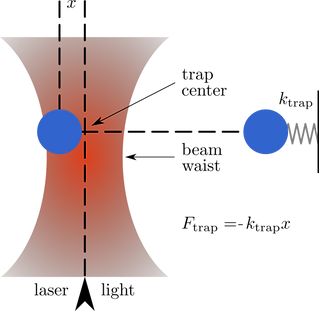top of page

# Projects

## Machine learning for strongly correlated materials

Although knowledge of phase transitions is generally obtained by studying order parameters, in some cases these might not be well defined or hard to measure (either experimentally or numerically).In this project we aim to understand if signatures of different phases of matter and crossovers can be detected using supervised and unsupervised learning techniques. In particular, how to extract valuable physics from projective measurements such as those used in quantum gas microscopes.

• Determinant Quantum Monte Carlo - How we do the physics

• Density Matrix Renormalization Group - How we do the physics

• Structural complexity - How we analyze the data and understanding the physics

## Interacting fermions with SU(N) symmetry

What do insulation, magnetism and superconductivity have in common? All of these diverse and interesting properties of materials arise from the behavior of electrons in a lattice.I seek to understand this behavior by stripping the system down to its very simple form named the Hubbard model, which accurately describes ultracold atomic systems that serve as quantum simulators of macroscopic materials.

• Universal thermodynamics of an SU(N) Fermi-Hubbard model

• Observation of antiferromagnetic correlations in an ultracold SU(N) Hubbard model

• Thermodynamics and magnetism in the 2D to 3D crossover of the Hubbard model

• Determinant Quantum Monte Carlo - How we do the physics

• Data cleaning - How we read the output and format it for easier manipulation

• Analysis and plotting - How we analyze data and generate figures

## Fermi Hubbard Optical Tweezer

Team Member

Although optical lattices have been of enormous use to simulate condensed matter systems, they are restricted to simple geometries such as squares.

In contrast, recent lattices formed by optical tweezer have the ability to not only construct a wide variety of geometries, but also have single-site control capabilities.I seek to numerical simulate the dynamics of atoms trapped in such tweezers, which are stroboscopically turned on and off while experiments are performed. The dynamics encode information such as the heating rate of the atoms in the lattice, which is relevant for experiments.

• Discrete Variable Representations (DVR) - How we diagonalize the matrices

• Time evolution - How we simulate the dynamics of the system

• Analysis and plotting - How we analyze data and generate figures

## Loop observables and classical Monte Carlo

Eventhough classical models do not suffer from the consequences of anticommutation and commutation relationships, computing the partition function and correlation functions is not a trivial task.

For this purpose, we employ classical Monte Carlo techniques to study lattice models. One of the most studied ones is the Ising model, which has an analytical solution in two dimensions, but not in three.We study generalizations of the Ising model in 3D with different lattice geometries and couplings. For this, we do not limit ourselves to n-point correlators, but loop observables, which depend on their topology, i.e. whether they are continuously shrinkable to a point, or non-shrinkable. Some if these models have an analytic solution for complex values of the coupling constants, and the existence of phases where these loop observables are quantized at fractional values. We aim to determine if similar phases can be found for all coupling constants that are all real.